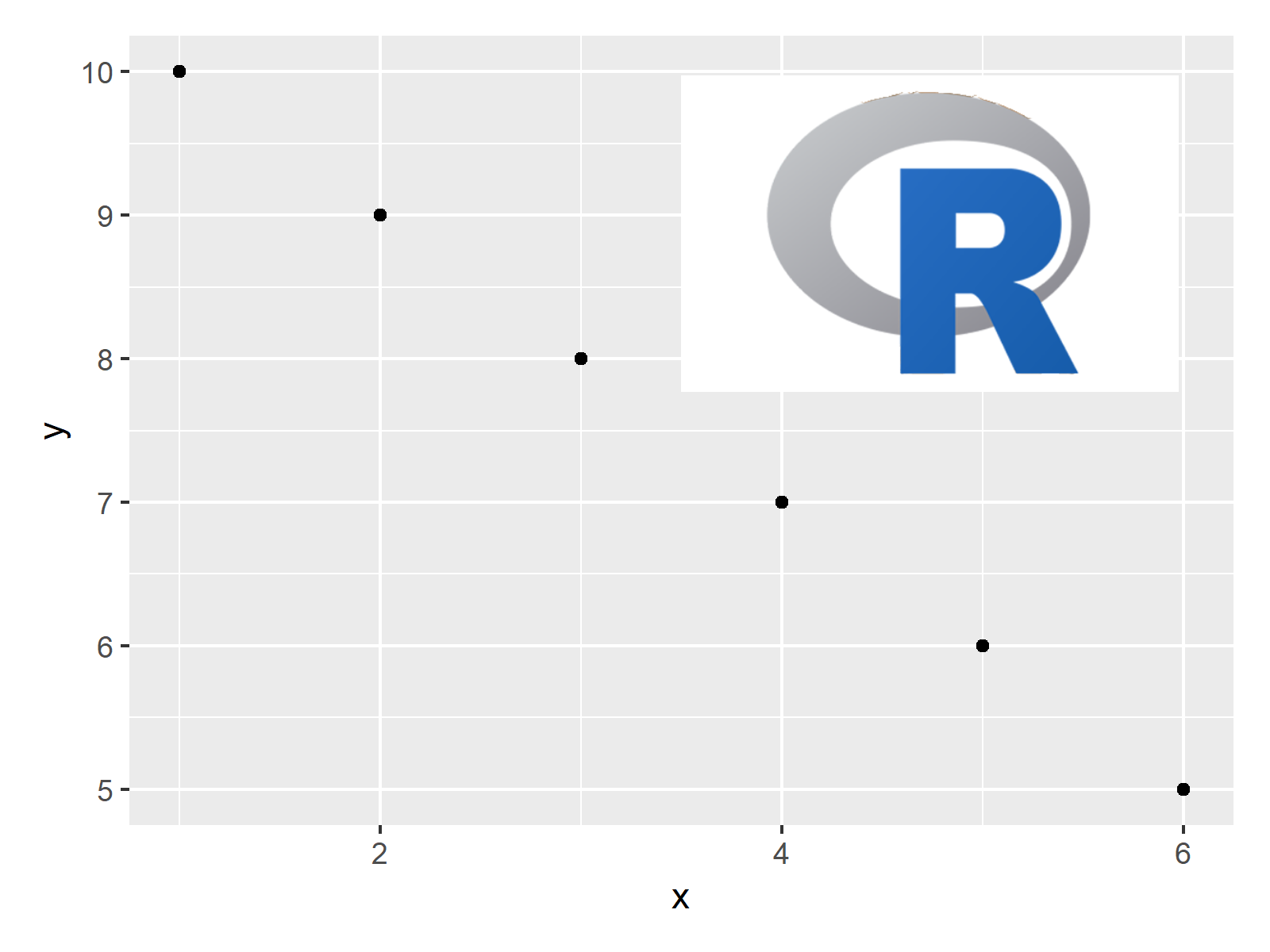# Add Image to Plot in R (Example) | Draw Picture on Top of Graph Using ggplot2 & patchwork Packages

In this tutorial, I’ll show how to add a picture on top of a ggplot2 plot in the R programming language.

The article consists of this content:

Let’s get started.

## Example Data, Add-On Packages & Default Graphic

First, let’s create some example data in R:

```data <- data.frame(x = 1:6,         # Creating example data
y = 10:5)
data                                # Printing example data
#   x  y
# 1 1 10
# 2 2  9
# 3 3  8
# 4 4  7
# 5 5  6
# 6 6  5```

Have a look at the previous output of the RStudio console. It shows that our example data has six rows and two numeric columns x and y.

We need to install and load the ggplot2 package, if we want to use the functions that are contained in the package:

```install.packages("ggplot2")         # Install ggplot2 package

Next, we can draw our data in a basic ggplot2 without an image:

```ggp <- ggplot(data, aes(x, y)) +    # Create ggplot2 plot
geom_point()
ggp                                 # Draw ggplot2 plot```Figure 1 shows the output of the previous syntax – A ggplot2 scatterplot with default specifications and without showing an image on top.

## Example: Add Image to Plot Using inset_element() Function of patchwork Package

In this example, I’ll show how to draw a ggplot2 plot with an image on top.

We first need to load an image to R using the png package. For this, we have to install and load the png package first:

```install.packages("png")             # Install png package

Now, we can use the readPNG function to load an image that we have stored on our computer:

`my_image <- readPNG("C:/Users/Joach/Desktop/r-logo.png", native = TRUE)`

If we want to add this image on top of our ggplot2 plot, we have to install and load the patchwork package as well:

```install.packages("patchwork")       # Install patchwork package

Now, we can use the inset_element function to add our picture to our ggplot2 graphic:

```ggp_image <- ggp +                  # Combine plot & image
inset_element(p = my_image,
left = 0.5,
bottom = 0.55,
right = 0.95,
top = 0.95)
ggp_image                           # Draw combined plot```The output of the previous R syntax is shown in Figure 2: A ggplot2 plot and an image on top.

## Video & Further Resources

Do you need further information on the R syntax of this post? Then I can recommend to watch the following video of my YouTube channel. I show the R programming codes of this article in the video.

Please accept YouTube cookies to play this video. By accepting you will be accessing content from YouTube, a service provided by an external third party.If you accept this notice, your choice will be saved and the page will refresh.

Furthermore, you may have a look at the other articles which I have published on my website. You can find some articles below:

In summary: In this tutorial you learned how to insert and combine plots and images in the same graph in the R programming language. In case you have additional questions, please let me know in the comments below.

Subscribe to the Statistics Globe Newsletter

•Koen Venema
May 9, 2023 8:46 pm

Hi Joachim,
Have been following your posts on Twitter for a while now. They are great and really helpful!
Based on this “Add Image to Plot in R”, I was wondering whether it would also be possible to plot another ggplot-graph (as an inset or something, or perhaps a zoom-in of the bigger plot, just brainstorming here…) on top of a larger ggplot, not as a png, but as a modifiable ggplot…
Does patchwork allow that too?
regards,
Koen

•May 12, 2023 2:21 pm

Hello Koen,

Yes, it is indeed possible to add another ggplot as an inset to a larger ggplot, and the patchwork package makes this process much simpler. You can create two separate ggplot objects and then add them together using the patchwork syntax. Here’s a simple example:

```# Load necessary packages
library(ggplot2)
library(patchwork)

# Create two ggplot objects
p1 <- ggplot(mtcars, aes(mpg, disp)) +
geom_point() +
theme_classic()

p2 <- ggplot(mtcars, aes(hp, wt)) +
geom_point() +
theme_classic()

p1 + inset_element(p2, left = 0.65, bottom = 0.65, right = 0.95, top = 0.95)```

If you want to create a zoom-in of a specific part of the larger plot, you might need to create a second plot that focuses on that specific area. See the following.

```# Create zoomed in plot
p3 <- ggplot(subset(mtcars, mpg > 15 & mpg < 20 & disp > 200 & disp < 300), aes(mpg, disp)) +
geom_point() +
theme_classic()

# Add the zoomed in plot as an inset
p1 + inset_element(p3, left = 0.65, bottom = 0.65, right = 0.95, top = 0.95)```

I hope you find the solutions helpful. Also, thank you for your nice feedback!

Regards,
Cansu